Next: Defining Compound Functions Up: Getting Started With Previous: Defining and Manipulating

## Defining Functions

Maple makes a strong distinction (as it should) between expressions and functions. An expression is made up of variables, numbers, and the standard mathematical operations of addition, subtraction, multiplication, division, and exponentiation. For example,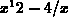is an expression, but x=2 is not, because the equals sign is not one of the allowed operations.

A function, on the other hand, is a more abstract object. It has a set of allowed input values and a rule for determining exactly one output value from each input values. That is, a function is an input-output machine. In practice, however, expressions are very often used to define functions, for example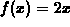, but there can be functions defined without using expresssions.

The syntax for defining functions in Maple uses the symbol ``->'', made by typing the two characters - and >. Using this syntax, the function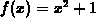is defined as follows.

```  > f:= x ->  x^2+1;
```Functions are handled differently than expressions are in Maple. You can evaluate the function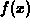at x=a with the simple command

> f(a);

You can also compose functions or define new functions in terms of old ones. Some examples are given below. Note that the independent variable you use to define the function is only a placeholder. That is, if you define a function f using x as the independent variable, Maple will cope with changing the independent variable in a way that is mathematically correct.

```  > f(2);
``````  > f(3*t);
```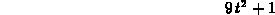```  > h := t -> sin(2*t);
```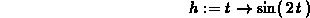```  > h(0);
```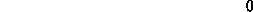```  > h(x);
``````  > f(h(t));
```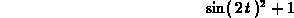```  > f(h(x));
```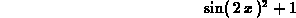William W. Farr
Mon Aug 28 09:31:56 EDT 1995